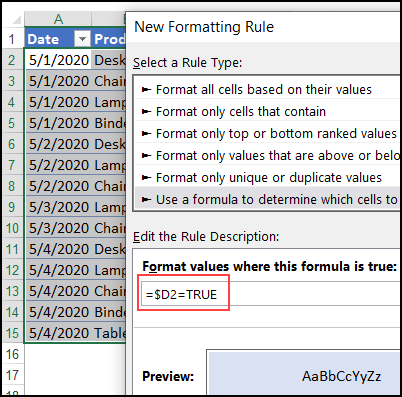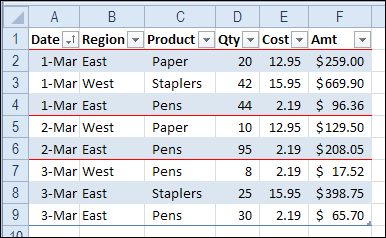## Colour Bands in Excel Table Based on Dates

Written by Debra Dalgleish from Contextures Blog

When you create a named table in Excel, you can colour the alternating rows with one of the built-in Table Styles. But how could you colour alternating groups of information, such as dates? This example shows show to create colour bands, based on dates, so it’s easy to see where each day’s data begins and ends.

### Colour Bands in Excel

In the steps shown below, you’ll see how to use Excel conditional formatting to create colour bands, based on the data in one column..

In this example, the sales rows for the dates are in alternating colours – blue and no fill. This technique was adapted from Chip Pearson’s site.First, we need to add a new column to the table, where a formula will check the date, and compare it with the date in the previous row.

• In cell D1, type a heading for a new column – TRUE
• In cell D2, enter this formula, to compare the dates:
• =IF(A1=A2,D1,NOT(D1))
• Press Enter, and the formula automatically fills down to the end of the table, with a result of TRUE or FALSE in each row.### How the Formula Works

The formula in cell D2 compares the date in column A, to the date in the cell above that

=IF(A1=A2,D1,NOT(D1))

If the dates are the same, the result is the value from column D, in the previous row

=IF(A1=A2,D1,NOT(D1))

If the dates are different, the result is the opposite of the value in the row above, because the Excel NOT function reverses TRUE and FALSE.

=IF(A1=A2,D1,NOT(D1))

In cell D2, the result is FALSE (the opposite of the TRUE in cell D1), because the date in cell A1 is not equal to the “Date” heading in cell A1.

NOTE: You could use either TRUE or FALSE as the heading in column D

### Table Style Optons

Before you add the conditional formatting, turn off banded rows in your Excel table, if that feature is active.

• Select a cell in the table
• On the Excel Ribbon, click the Table Design tab
• In the Table Style Options group, remove the check mark for Banded Rows.Then, follow these steps to add the conditional formatting that creates colour bands:

• Starting  from row 2, select all the data cells in the table
• On the Home tab, click Conditional Formatting, New Rule
• Click on “Use a formula to determine which cells to format”
• In the formula box, type this formula, referring to the active data cell:
• =\$D2=TRUE
• Click the Format button, and choose a fill colour for the rows that have TRUE in column D
• Click OK, twice, to apply the formatting
• (Optional) Hide the TRUE/FALSE column, to tidy up the worksheet.### Absolute Reference

In the conditional formatting rule, =\$D2=TRUE, we use an absolute reference to column D (\$D), instead of a relative reference (D).

• With an absolute reference, all the columns will refer to the value in column D
• With a relative reference, the formula would adjust in each column, and each cell would check its own value, instead of the cell in column D.

For example, the conditional formatting in column B would look for TRUE in column E, instead of column D. Column E is empty, so there’s no colour applied in column B.### Add a Border to Separate Groups

Another way to separate the groups is with a top border, like I did with this list of dates.

You don’t been an extra column for this technique.### Colour Row Based on One Cell’s Value

This video shows how to format multiple cells in a row, based on on cell’s value, using an absolute reference.

### More Conditional Formatting Examples

See more conditional formatting examples on my Contextures website, and download the sample file there.

_________________

### Colour Bands in Excel Table Based on Dates_________________

Colour Bands in Excel Table Based on Dates is a post from Contextures Blog and is not allowed to be copied to other sites